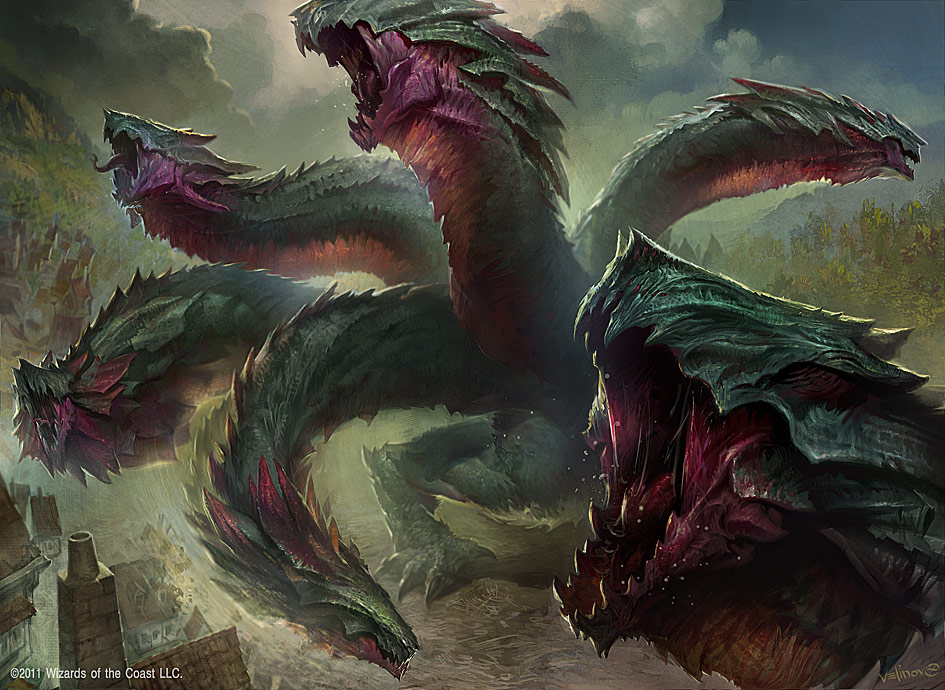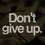# The Dark Eternal NightArtwork taken from DeviantArt.

Our magnificent friend Daniel Ploch inspired me to publish this note. My proposed problems are thus not very original, and clearly inspired by his The Hydra and by many of Dream Theater's songs.

Before a sword-armed hero stands a mythological n-Hydra. It begins, graciously enough, with but one head. The hero has one way to attack the beast - slice off its head.

But this is no sure method, for, with equal probabilities, a severed head of the Hydra may either wither into nothing... or sprout again as $n$ new different heads.

Way off in the distance, stands a wizard, watching the combat through his looking glass. He is, however, very bored of watching the epic combat, since our hero can only slice one hydra-head per minute, leaving the magician with nothing but an incredible desire to sleep. Our spectator is blood-thirsty though, and randomly wakes from his naps from time to time, counting the many defeated and alive heads of the myth beast.

Let $P_n$ be the probability that the hero defeats the n-Hydra.

Let $T$ be the time, in minutes, since the combat began at $T = 0$.

Consider that the hero only takes action on integer times of $T$, and so does the wizard.

Remember, $n$ is always a positive integer.

Level 1: Show that victory is always certain for $n = 1$.

Level 2: Show that victory is always certain for $n= 2$, although it may sound counter-intuitive.

Level 3: Show that $\displaystyle \lim_{n \to \infty} P_n = \dfrac{1}{2}$.

Level 4: Consider that the wizard takes a full-combat nap, waking up only after the end of the n-Hydra's existence. How many dead heads is he expected to find? Display the numerical result for $n =3$ and find a formula for a generic value of $n$.

Level 5: Evaluate, for a generic value of $n$, the expected number of $D$ dead n-Hydra heads and $A$ alive heads counted by the wizard throughout integer values of $T > 0$. Consider that the wizard immediately wakes up and leaves if the last Hydra head is defeated.Note by Guilherme Dela Corte
6 years, 3 months ago

This discussion board is a place to discuss our Daily Challenges and the math and science related to those challenges. Explanations are more than just a solution — they should explain the steps and thinking strategies that you used to obtain the solution. Comments should further the discussion of math and science.

When posting on Brilliant:

• Use the emojis to react to an explanation, whether you're congratulating a job well done , or just really confused .
• Ask specific questions about the challenge or the steps in somebody's explanation. Well-posed questions can add a lot to the discussion, but posting "I don't understand!" doesn't help anyone.
• Try to contribute something new to the discussion, whether it is an extension, generalization or other idea related to the challenge.
• Stay on topic — we're all here to learn more about math and science, not to hear about your favorite get-rich-quick scheme or current world events.

MarkdownAppears as
*italics* or _italics_ italics
**bold** or __bold__ bold
- bulleted- list
• bulleted
• list
1. numbered2. list
1. numbered
2. list
Note: you must add a full line of space before and after lists for them to show up correctly
paragraph 1paragraph 2

paragraph 1

paragraph 2

[example link](https://brilliant.org)example link
> This is a quote
This is a quote
    # I indented these lines
# 4 spaces, and now they show
# up as a code block.

print "hello world"
# I indented these lines
# 4 spaces, and now they show
# up as a code block.

print "hello world"
MathAppears as
Remember to wrap math in $$ ... $$ or $ ... $ to ensure proper formatting.
2 \times 3 $2 \times 3$
2^{34} $2^{34}$
a_{i-1} $a_{i-1}$
\frac{2}{3} $\frac{2}{3}$
\sqrt{2} $\sqrt{2}$
\sum_{i=1}^3 $\sum_{i=1}^3$
\sin \theta $\sin \theta$
\boxed{123} $\boxed{123}$

## Comments

Sort by:

Top Newest

Here's a way at looking at it:

Consider the expected value of the number of heads that appear each turn.

Given any head that is chopped off, it has a $\dfrac{1}{2}$ probability of dying and a $\dfrac{1}{2}$ probability of turning into $n$ heads. Thus, the expected number of heads to appear is $\dfrac{n}{2}$ per 1 head chopped off.

Thus, the number of heads expected to appear after $k$ heads are chopped off is $\dfrac{kn}{2}$.

When $\dfrac{kn}{2} > k$, or $n > 2$, then the expected number of heads to regrow after getting rid of all existing heads is larger than the number of existing heads before the warrior cut them, so on average the case is hopeless.

Thus, the only way to kill the hydra is to lop its head off the first go, which has probability $\dfrac{1}{2}$.

- 6 years, 3 months ago

Log in to reply

That answers L3 intuitively. Can you post a pure algebraic solution?

Also, proceed with the expected value reasoning to answer L4 and L5.

- 6 years, 3 months ago

Log in to reply

×

Problem Loading...

Note Loading...

Set Loading...